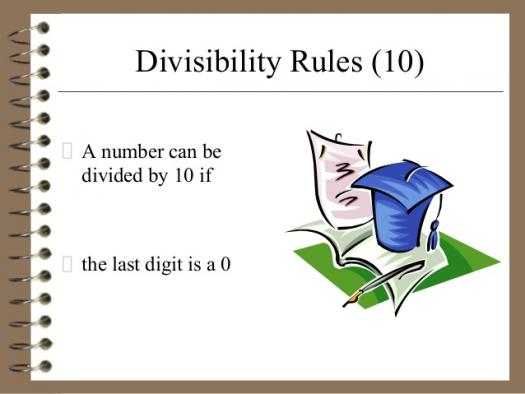# What Do You Know About Divisibility Tests?

Approved & Edited by ProProfs Editorial Team
At ProProfs Quizzes, our dedicated in-house team of experts takes pride in their work. With a sharp eye for detail, they meticulously review each quiz. This ensures that every quiz, taken by over 100 million users, meets our standards of accuracy, clarity, and engagement.
| Written by Livyn
L
Livyn
Community Contributor
Quizzes Created: 348 | Total Attempts: 140,973
Questions: 10 | Attempts: 683SettingsA divisibility rule is a shorthand way of determining whether a given integer is divisible by a fixed divisor without performing the division, usually by examining its digits. Although there are divisibility tests for numbers in any radix, or base, and they are all different, this article presents rules and examples only for decimal, or base 10, numbers. Martin Gardner explained and popularized these rules in his September 1962 "Mathematical Games" column in Scientific American.

• 1.

### Who explained and popularized divisibility rules?

• A.

Martin Gardner

• B.

Abraham Lincoln

• C.

Isaac Newton

• D.

Albert Einstein

A. Martin Gardner
Explanation
Martin Gardner is the correct answer because he was a popular American mathematics and science writer who explained and popularized many mathematical concepts, including divisibility rules. He wrote numerous books and articles on recreational mathematics, making complex mathematical ideas accessible and enjoyable for a wide audience. His work had a significant impact on the popularization and understanding of mathematics, making him a well-known figure in the field.

Rate this question:

• 2.

### Martin Gardner explained and popularized these rules in?

• A.

June 1997

• B.

September 1962

• C.

July 1999

• D.

May 1964

B. September 1962
Explanation
In September 1962, Martin Gardner explained and popularized these rules.

Rate this question:

• 3.

### Divisibility by seven can be tested by what?

• A.

• B.

Subtraction

• C.

Multiplication

• D.

Division

C. Multiplication
Explanation
Divisibility by seven can be tested by multiplication. This is because there is a rule that states if a number is divisible by seven, then its multiples are also divisible by seven. By multiplying a given number by different integers, we can check if the resulting numbers are divisible by seven. If they are, then the original number is also divisible by seven.

Rate this question:

• 4.

### The Pohlman–Mass method provides a quick solution that can determine if most integers are divisible by .....?

• A.

Seven in three steps or less

• B.

Four in two steps or less

• C.

Six in three steps or more

• D.

Eight in two steps or less

A. Seven in three steps or less
Explanation
The Pohlman-Mass method is a quick solution to determine if most integers are divisible by seven in three steps or less. This method is efficient and can be used to quickly identify if a number is divisible by seven without having to perform lengthy calculations.

Rate this question:

• 5.

### Divisibility by 6 is determined by checking the original number to see if it is both an ....?

• A.

Odd number

• B.

Even number

• C.

Denominator number

• D.

Numeral number

B. Even number
Explanation
Divisibility by 6 is determined by checking if the original number is both an even number and divisible by 3. This is because 6 is a multiple of both 2 and 3. If a number is even, it is divisible by 2, and if it is divisible by 3, it will also be divisible by 6. Therefore, for a number to be divisible by 6, it must be an even number.

Rate this question:

• 6.

### Divisibility by 5 is easily determined by checking the last digit in the number (475), and seeing if it is either ....?

• A.

0 or 1

• B.

2 or 4

• C.

1 or 3

• D.

0 or 5

D. 0 or 5
Explanation
Divisibility by 5 is easily determined by checking the last digit in the number. If the last digit is either 0 or 5, then the number is divisible by 5. This is because any number that ends in 0 or 5 is a multiple of 5. Therefore, the correct answer is 0 or 5.

Rate this question:

• 7.

### If the last number is either 0 or 5, the entire number is divisible by ....?

• A.

5

• B.

4

• C.

3

• D.

2

A. 5
Explanation
If the last digit of a number is either 0 or 5, it means that the number is divisible by 5. This is because any number ending in 0 or 5 is a multiple of 5. Therefore, the entire number is divisible by 5.

Rate this question:

• 8.

### Also, in divisibility by 5, if the last digit in the number is 0, then the result will be the remaining digits multiplied by ...?

• A.

1

• B.

2

• C.

4

• D.

3

B. 2
Explanation
In divisibility by 5, if the last digit in the number is 0, then the result will be the remaining digits multiplied by 2. This is because any number that ends with 0 is divisible by 5, and when we divide it by 5, the result will be the remaining digits multiplied by 2.

Rate this question:

• 9.

### No special condition. Any integer is divisible by ...?

• A.

1

• B.

6

• C.

7

• D.

2

A. 1
Explanation
Any integer is divisible by 1 because division by 1 results in the number itself. In other words, any number divided by 1 equals the same number. Therefore, any integer can be evenly divided by 1 without leaving a remainder.

Rate this question:

• 10.

### Divisibility rule is a subject under which course?

• A.

Biology

• B.

Maths

• C.

Music

• D.

GovernmentBack to top